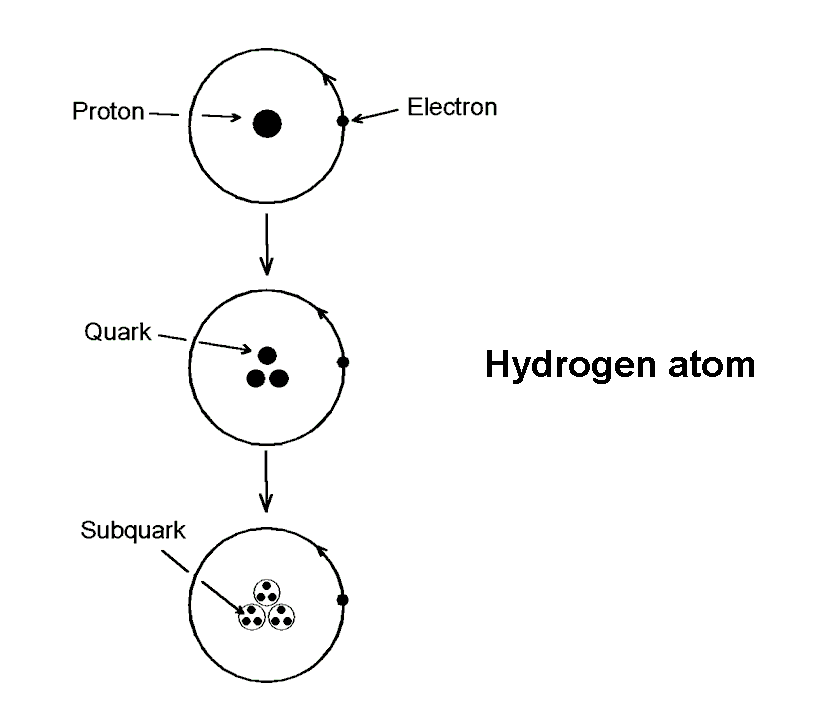<< Previous    1    3  4  5  ...50    Next >>The simplest atom is the hydrogen atom. The positively charged proton is at its centre. One negatively charged electron orbits it, bound by their mutual electromagnetic attraction. The diagram opposite depicting this is purely schematic, as the electron does not move in a well-defined circle.

The proton consists of two up down quarks with electric charge +(2/3)e and one down quark with electric charge –(1/3)e, where e is the magnitude of the charge carried by the electron. The three quarks are bound together by exchanging eight spin-1 particles called "gluons."

According to so-called "preon theories," quarks and leptons, such as the electron, are composite bound states of still smaller particles. According to the author, however, leptons are discrete particles and quarks are composed of three subquarks. As discussed in Occult Chemistry, this compositeness of quarks is the revolutionary implication of the micro-psi observations of Annie Besant & C.W. Leadbeater. It is not yet firmly confirmed by any experimental data of high-energy physics, although it is consistent with certain data, such as the Ι t Ι−2 behaviour of the proton magnetic form factor F(t) as Ι t Ι → ∞, where t is the four-momentum transfer squared*.

If quarks are, indeed, composed of three particles and electrons are single particles, the hydrogen atom consists of ten indivisible particles, namely, three triplets of subquarks in the proton and one electron that orbits the latter.

* "Composite quarks and hadron-lepton unification," S.M. Phillips, Physics Letters, Vol. 84B, number 1 (4 June, 1979), 133-136.

 << Previous    1    3  4  5  ...50    Next >>

Home